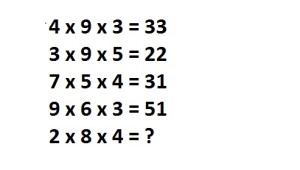Home > Math Shortcuts > Brain Trigger #14 – Sample Number puzzle question

# Brain Trigger #14 – Sample Number puzzle question

## Brain Trigger #14 – Sample Number puzzle question

Now here we will discuss few question answers on Number puzzle which are very common in competitive exams. These types of Number puzzle questions will help you to prepare for your exam. Using your math skills you can solve these questions by yourself. Shortcut tricks can also be used to solve these Number puzzle questions.

We try every types of Number puzzle questions and their shortcut methods to bring together here in this website. what you need to do is to apply these tricks in Number puzzle problem to solve. You can solve these questions without using any shortcut tricks also.

Now try to solve a question on Number puzzle. First of all you need to read the question very carefully and try to solve it by yourself. We provide the answer below. If you solve the question then check your answer with our solution. Don’t know how to do it? Check below for the solution of this question.

Each page is having a question on Number puzzle and also contain its solution with detail explanation. Next/Previous link will help you to navigate through other questions.

Let’s begin question with answer session. Today’s question is:

What number should replace the question mark ?To know the answer of this question please scroll down. You can take as much time as you want to solve the question. But the quickest you solve it the more you gain.

(Scroll down to get the answer)

(Scroll down to get the answer)

(Scroll down to get the answer)

(Scroll down to get the answer)

Now here we will discuss the answer of above question. If you already done this by yourself then please check your answer with us. If you correctly answer the question then Well Done, but don’t worry if you are not able to answer the question correctly. Solution with explanation of the following question is provided below.

You can solv this way……..

Replace all second multiplication sign with minus sign and you will get the answer of all

4 x 9 – 3 = 33
3 x 9 – 5 = 22
7 x 5 – 4 = 31
9 x 6 – 3 = 51
2 x 8 – 4 = 12

 << PREVIOUS QUESTION NEXT QUESTION >>

Few questions on some other topics are also provided here. Click on the Next and Previous link to get few more questions and answers.

1.